Deep convnets for image recognition
Deep Convolutional Networks

## Convolutional Neural Nets: Introduction¶

Translation Invariance

• Image
• Different positions
• Same objects
• Text
• Kitten in a long text
• You can use weight sharing and train them jointly for those inputs

Convnets

• Neural networks that share their parameters across space
•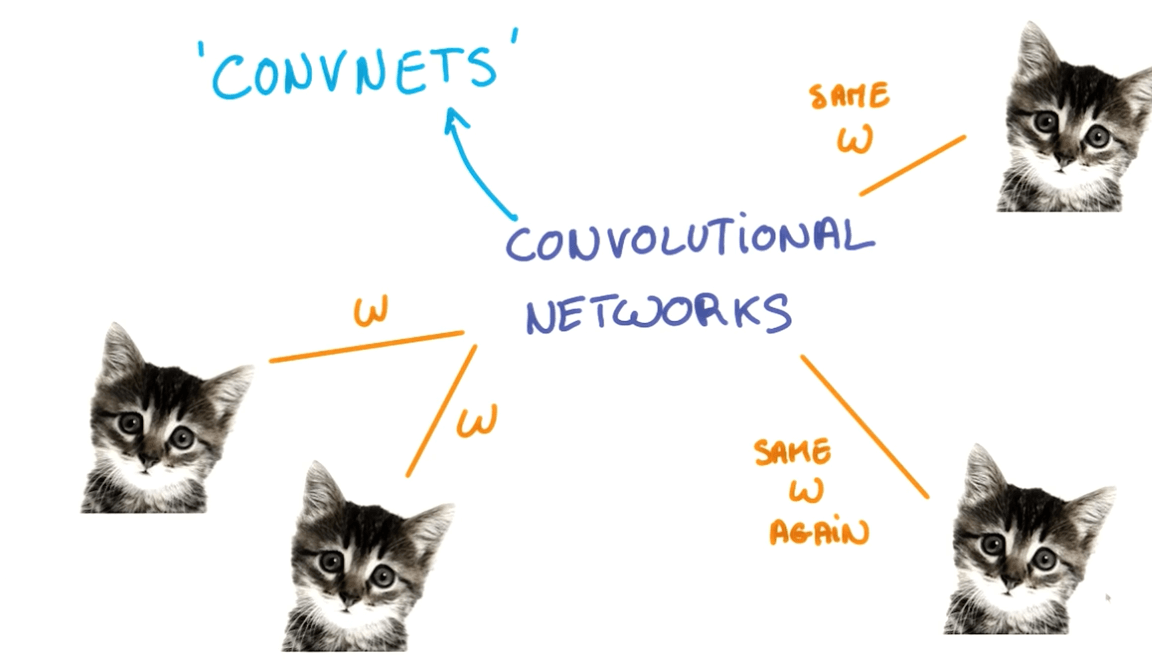• We take a portion of the image and run a neural network.
•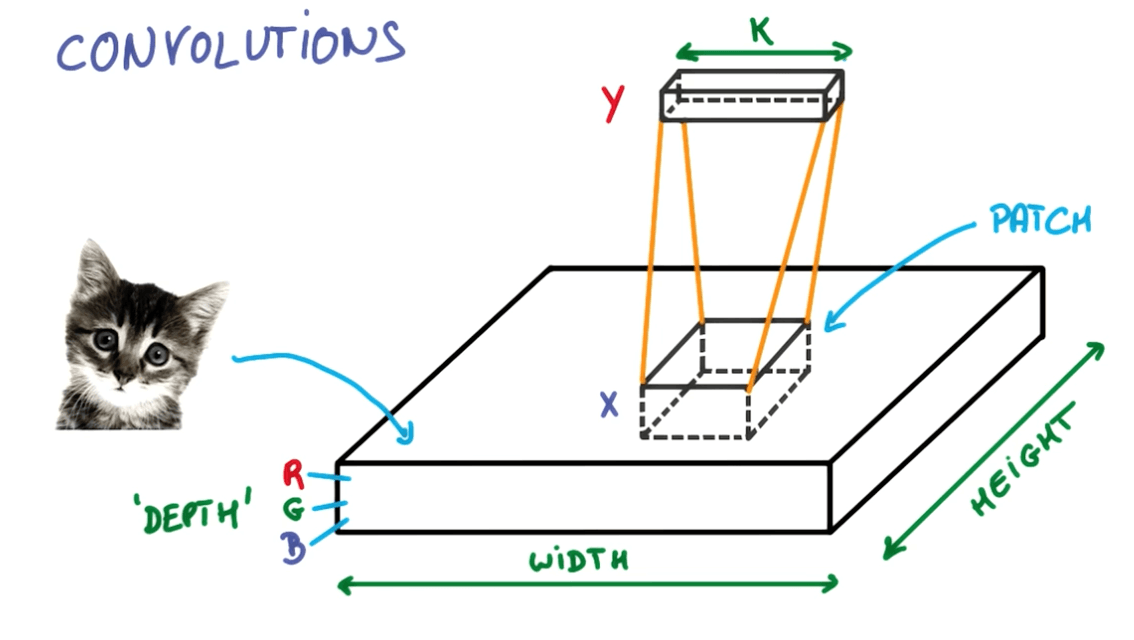•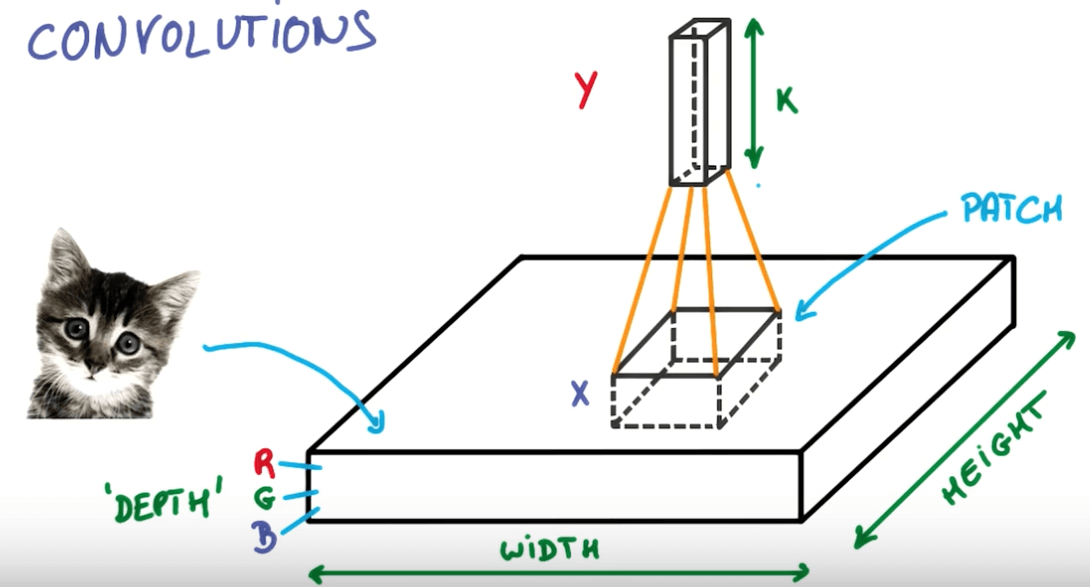• We then slide the neural network across the image
• Here you can see we've a layer that has a deeper depth but smaller space.
• We will slide the neural network on this layer that will again increase the depth and reduce the space.
•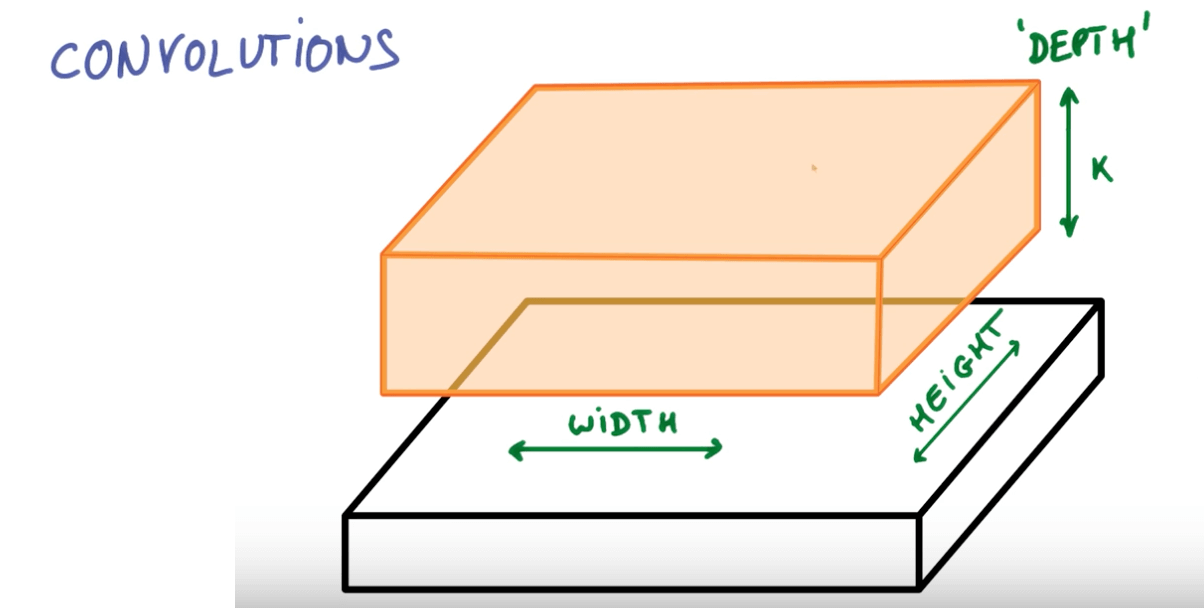• We continue to do this until we've reached a stage of maximum depth k where k are the outputs we want.
• Instead of having stacks of matrix multipliers, we would have stacks of convolutions.
•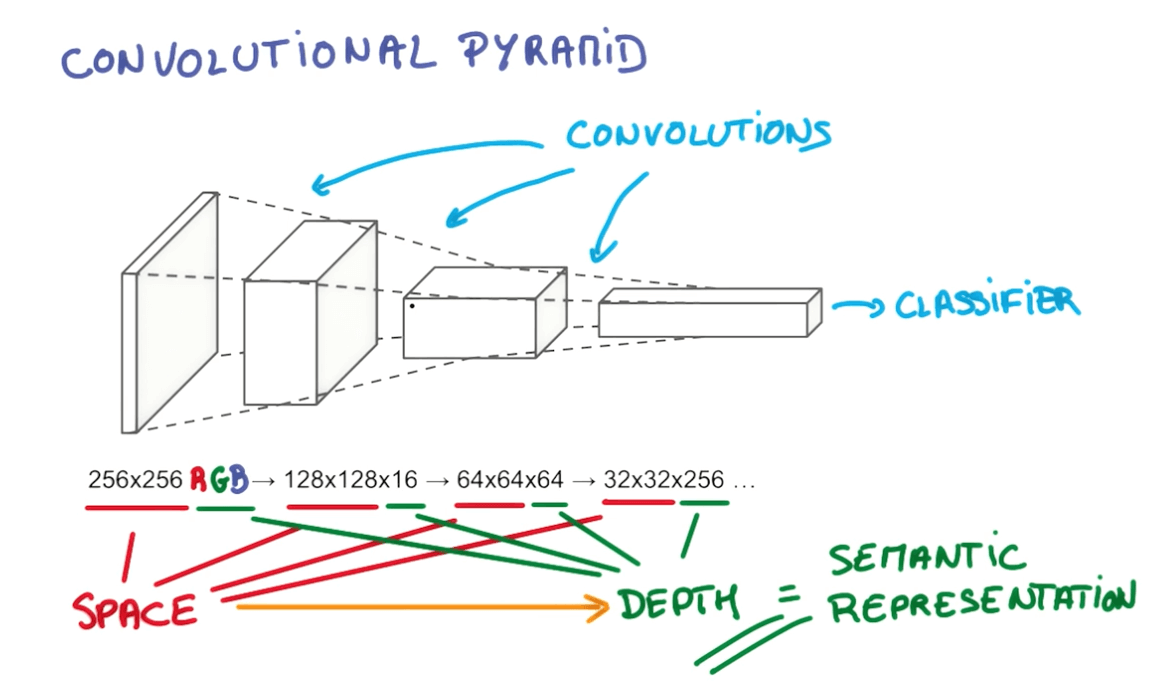• Here you can see we're trying to reduce the space and increase the depth.

Convnets Terms

•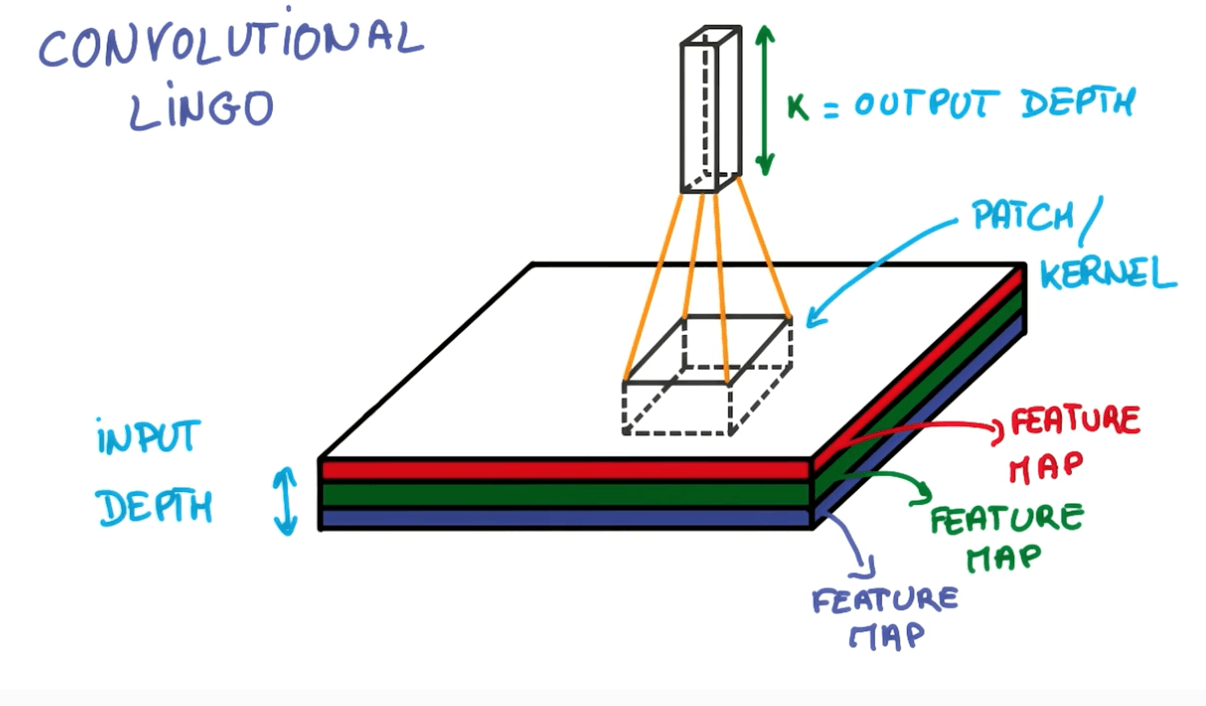• Strides
•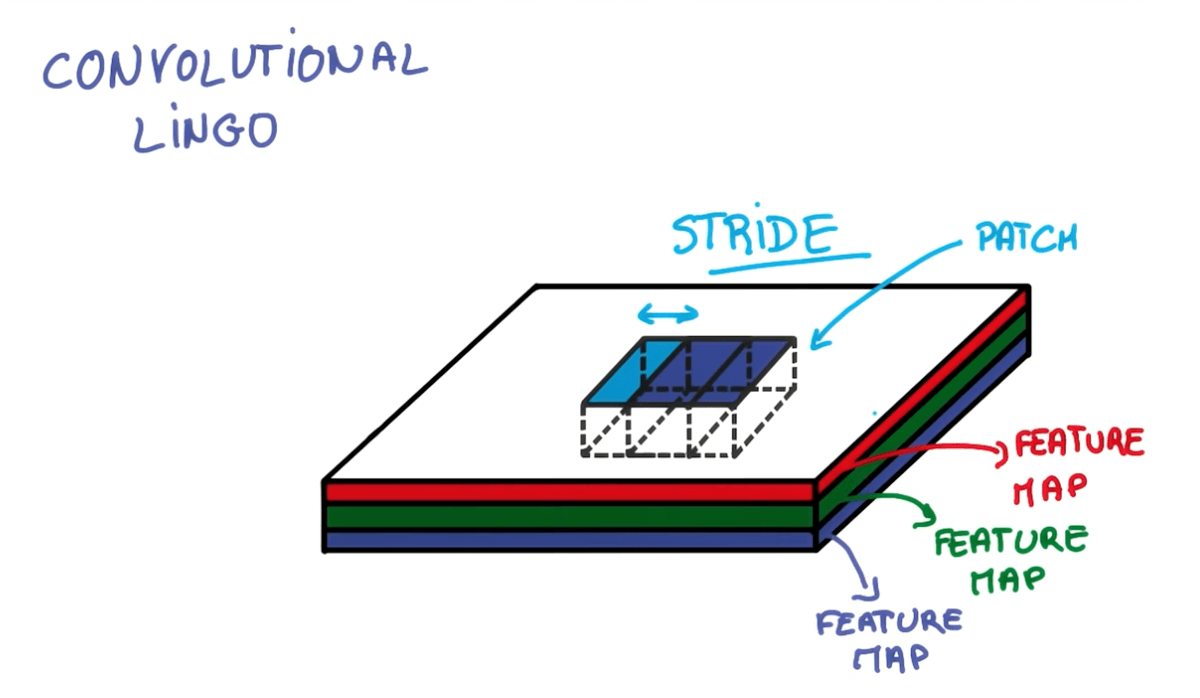• Where stride is the number of pixels that we are shifting.
• Stride: 1
• Output same size as input
•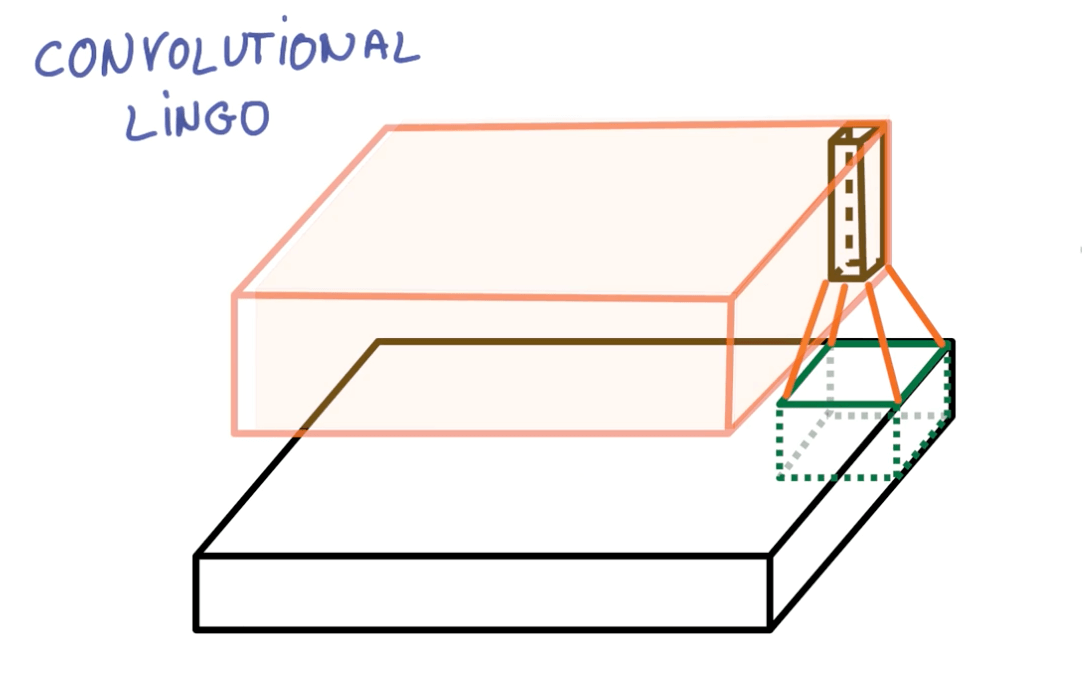• Stride: 2
• Output roughly half the size
•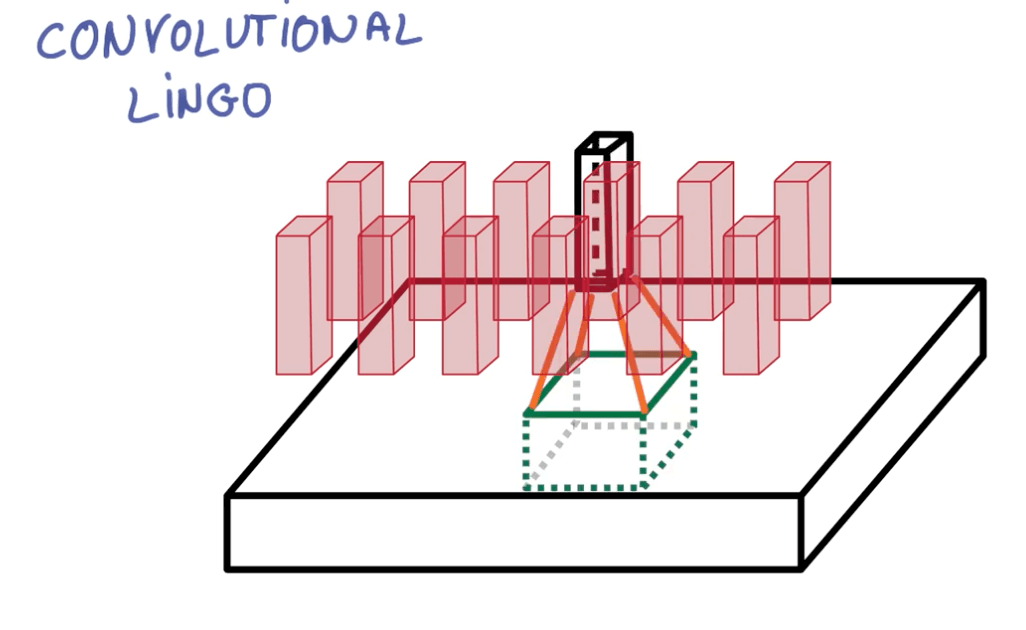•• Imagine you have 28x28 image.
• You run a 3x3 convolution on it.
• Input depth: 3
• Output depth: 8
•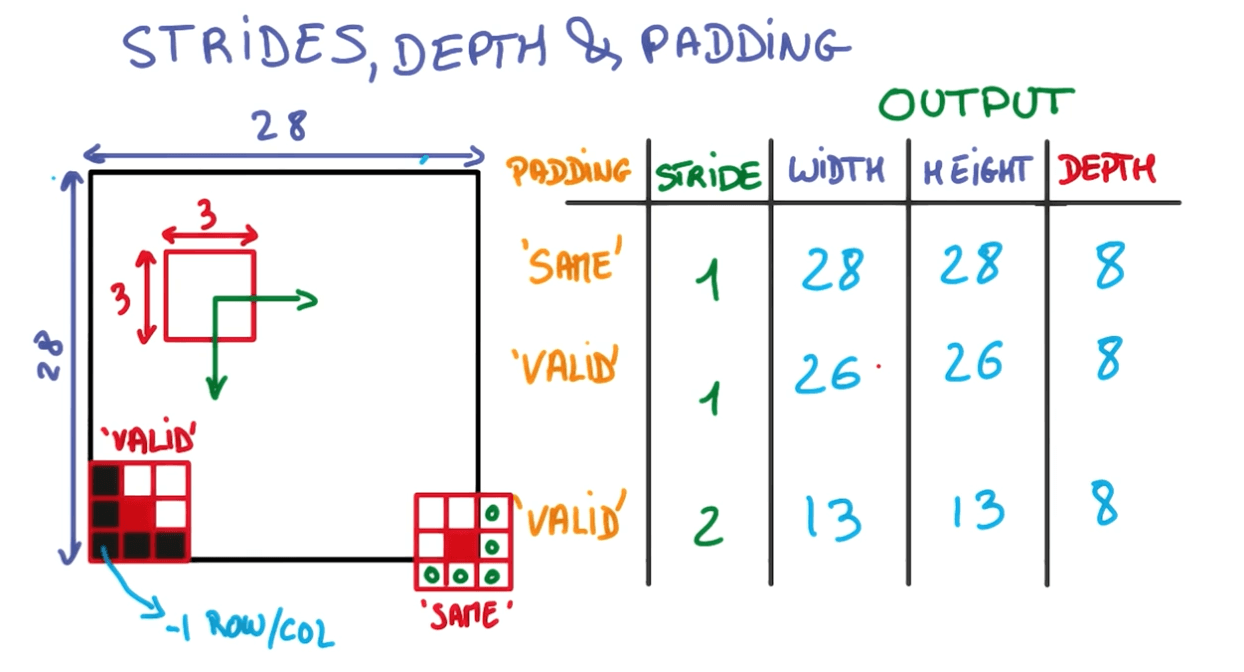• For stride: 1 and padding: same (1)
• You would have the exact same dimensions.
• You would be taking a F x F x D_input dot-product to come up with a number.
• For stride: 1 and padding: valid (0)
• You would have one less row and column
• For stride: 2 and padding: valid (0)
• You would have half the output.

Calculating Output Size

• $O = \frac {W - K - 2P} {S} + 1$
• O is the output height/length
• W is the input height/length
• K is the filter size (kernel size)
• S is the stride

• In general it's common to see same (zero) padding, stride 1 and filters of size FxF.
• Zero-padding = $\frac {F - 1}{2}$
• This might not be something you want.

Depth

• Number of filters = depth.
• We try to keep this in powers of two.
• 32, 64, 128, 512 etc.
• This is for computational reasons.

Number of Parameters

• Number of parameters in layer = (F x F x D_input + 1) x D_filter
• Where F is the filter size
• D_input is the depth of the input layer
• 1 is the bias
• D_filter is the depth of the filter
• Parameters per filter: (F x F x D_input + 1)

Convolution Networks

•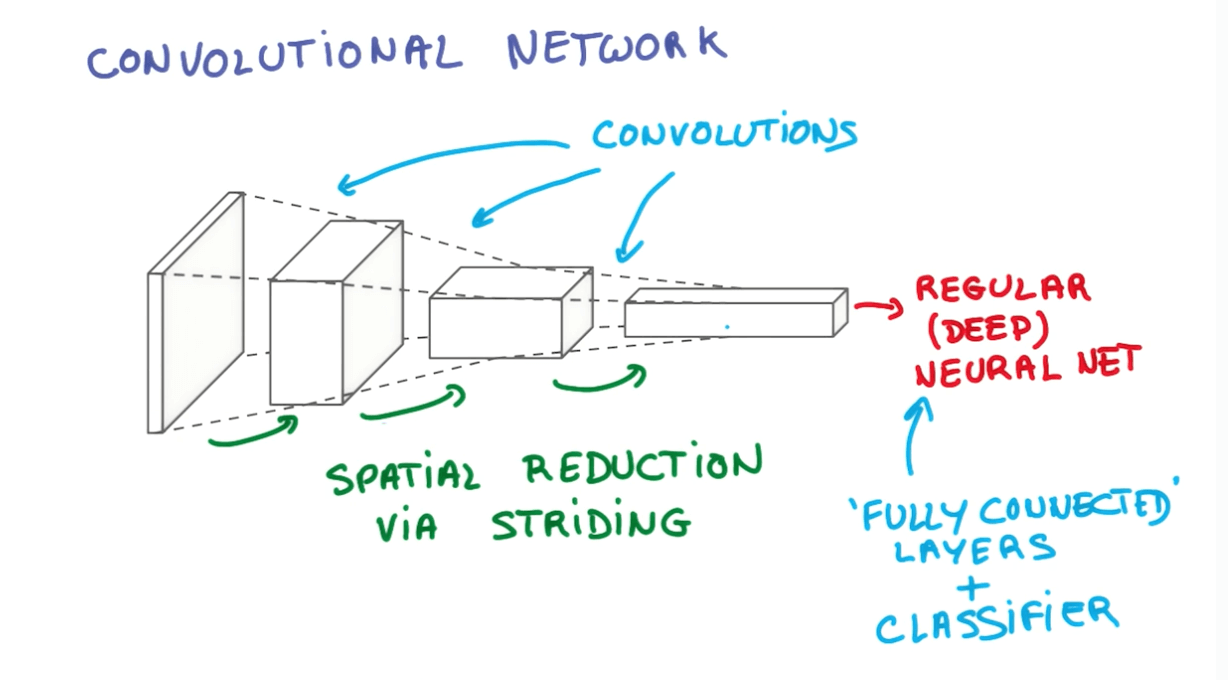•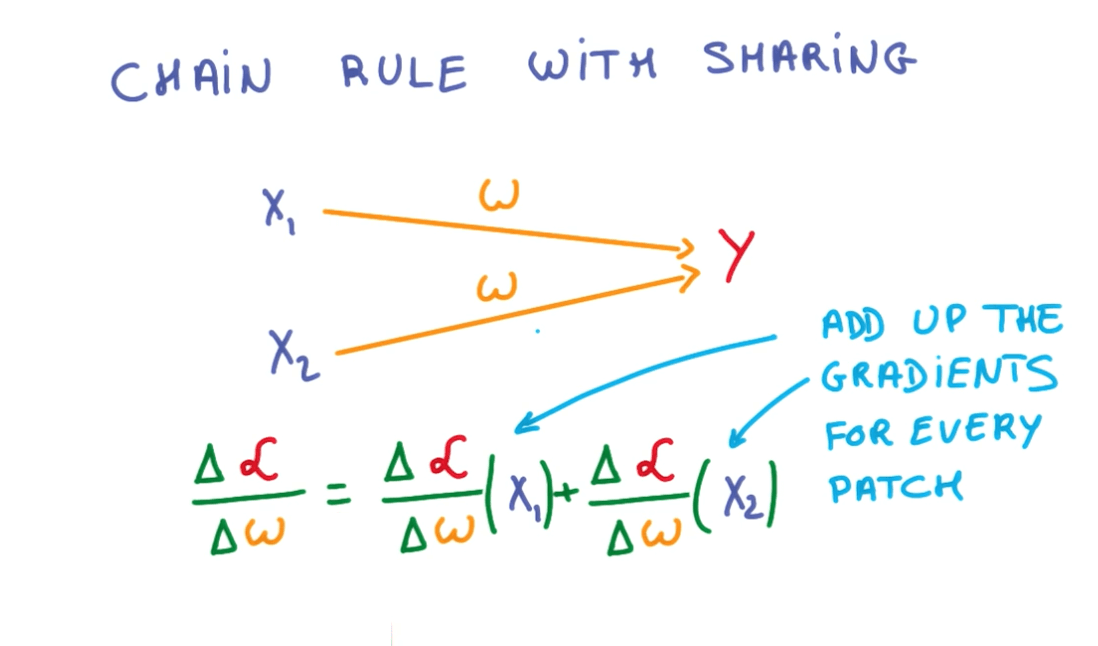• Fully Connected (FC) Layer
• Basically it connects to the entire input volume like a neural network.
• Final layer after we have done all our convolutions.
• ReLU Layers
• Remember there are ReLU Layers after every Conv and FC.

• Pooling
• 1 x 1 convolutions
• Inception

Pooling

• Striding
• We shift the filter by a few pixel each time.
• This is very aggressive method that removes a lot of information.
• Pooling
• We can take a smaller stride.
• Take all the convolutions in the neighbors.
• Combine them somehow, and this is called pooling.
• We will be preserving the depth.
• But we will be reducing the width and height.
•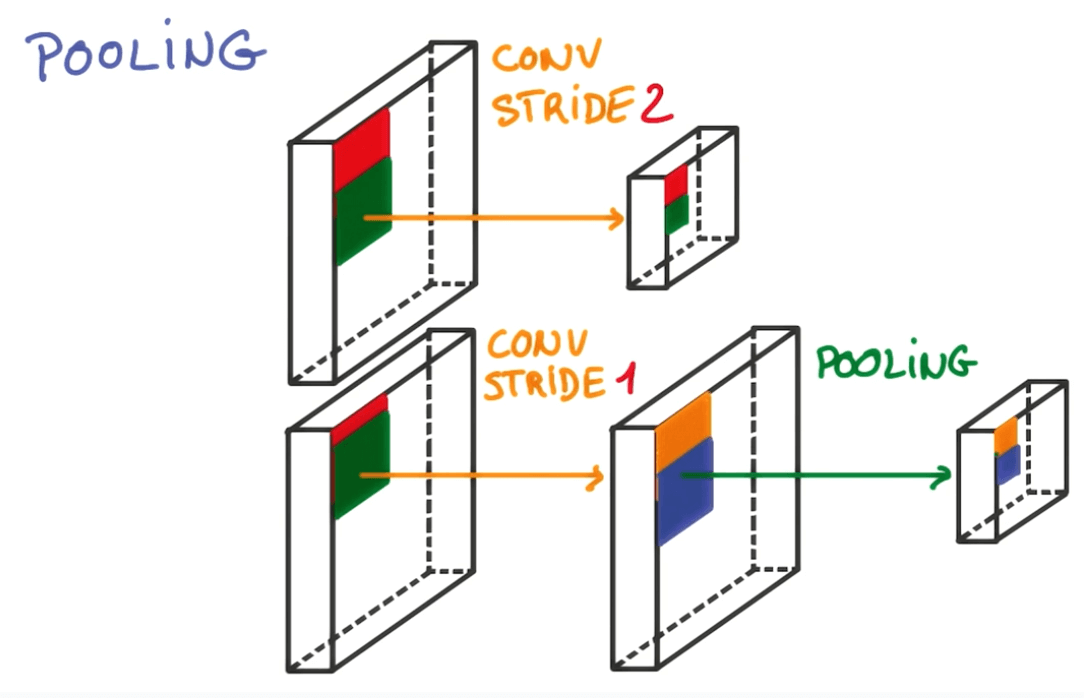1. Max Pooling
• At every point in a feature map, look at a small neighborhood around that point and compute the maximum of all the responses around it.
•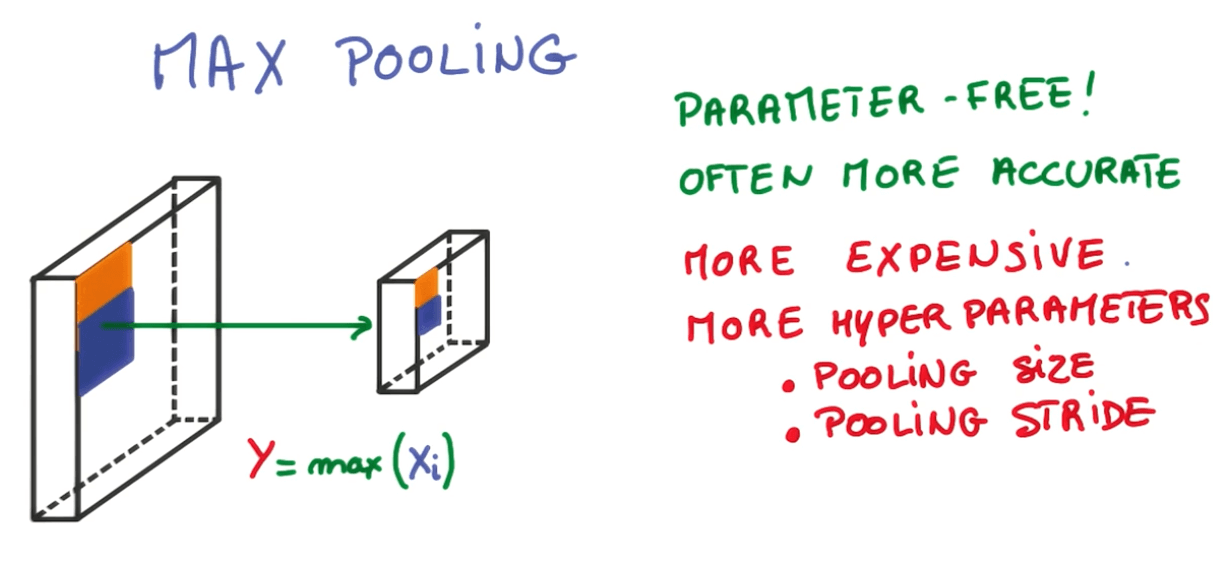• Typical architecture
•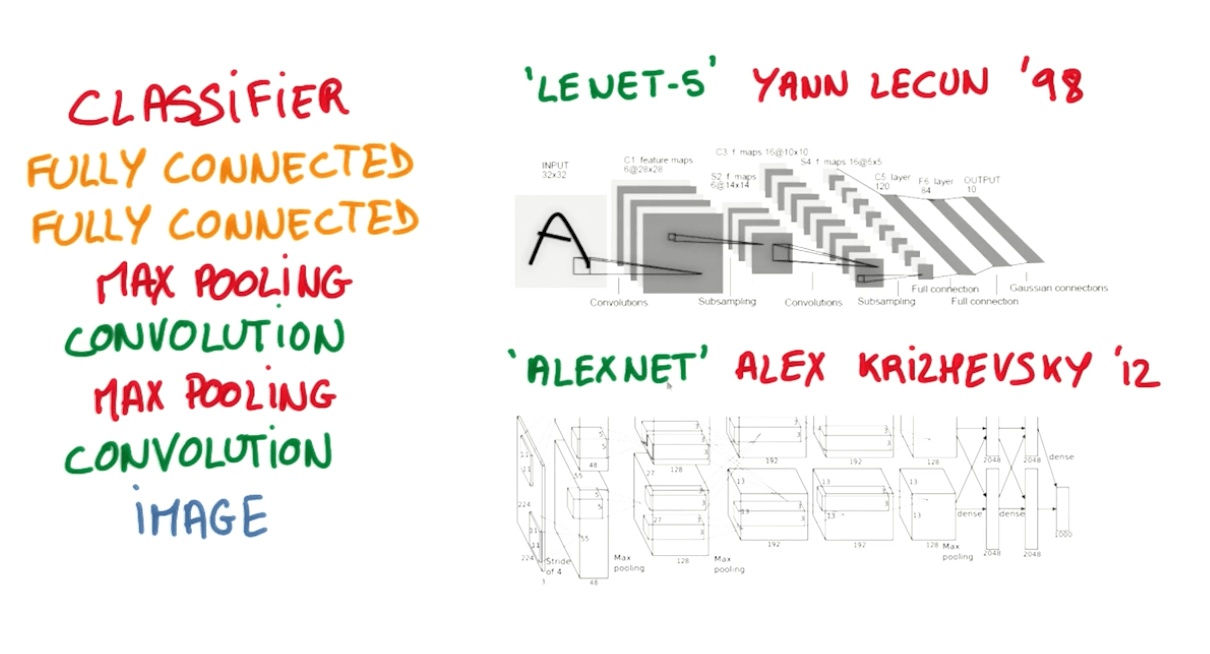1. Average pooling
• Instead oftaking the max, we take the average.
• It's similar to taking a blurred, low-resolution, view of the feature map.
•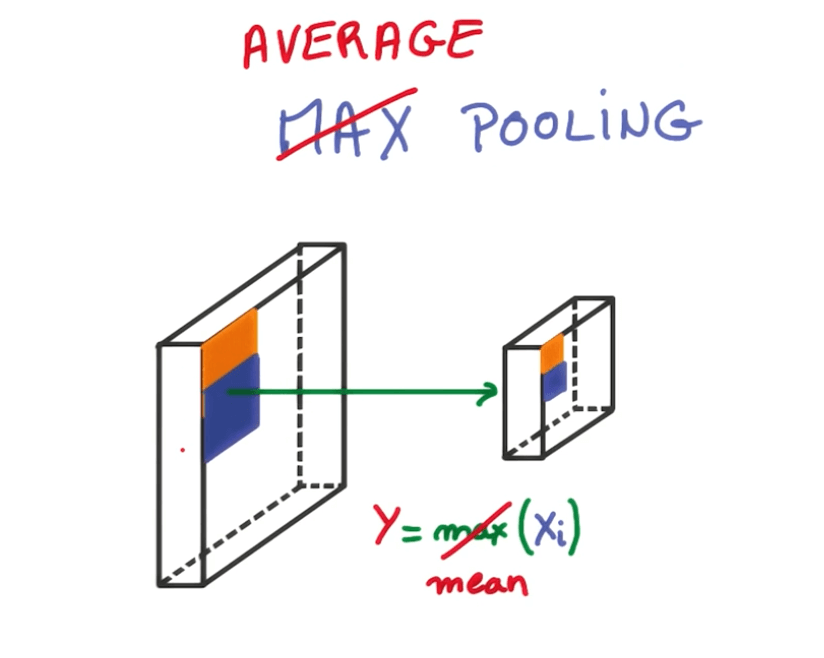1x1 Convolutions

• Here we are using only 1 pixel by 1 pixel.
•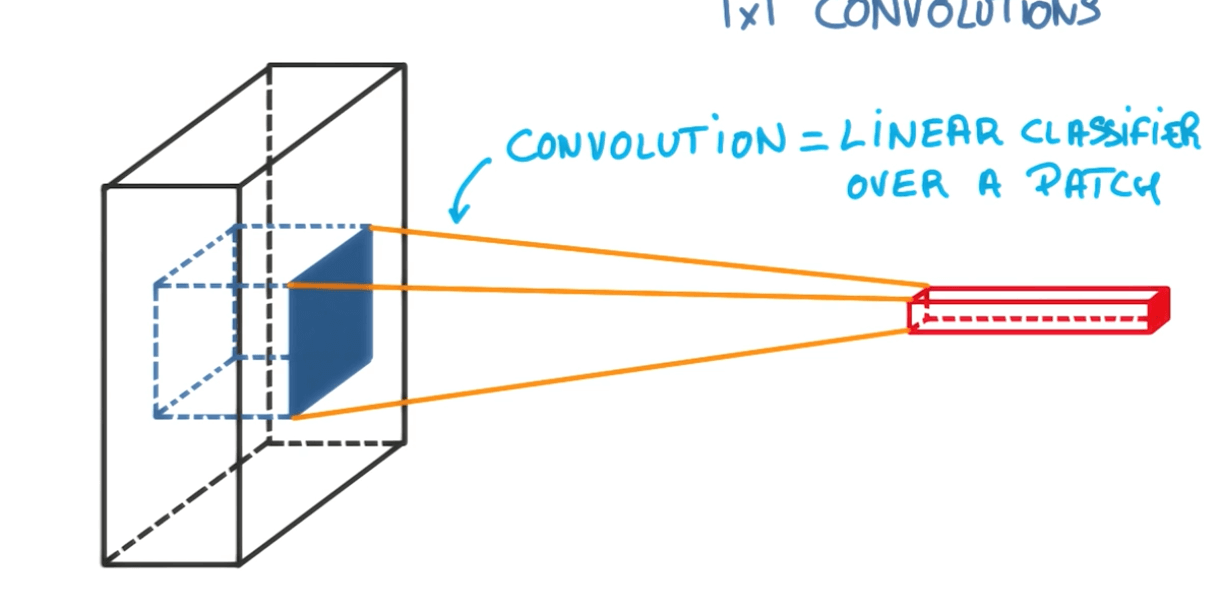• Now we add a 1x1 convolution.
•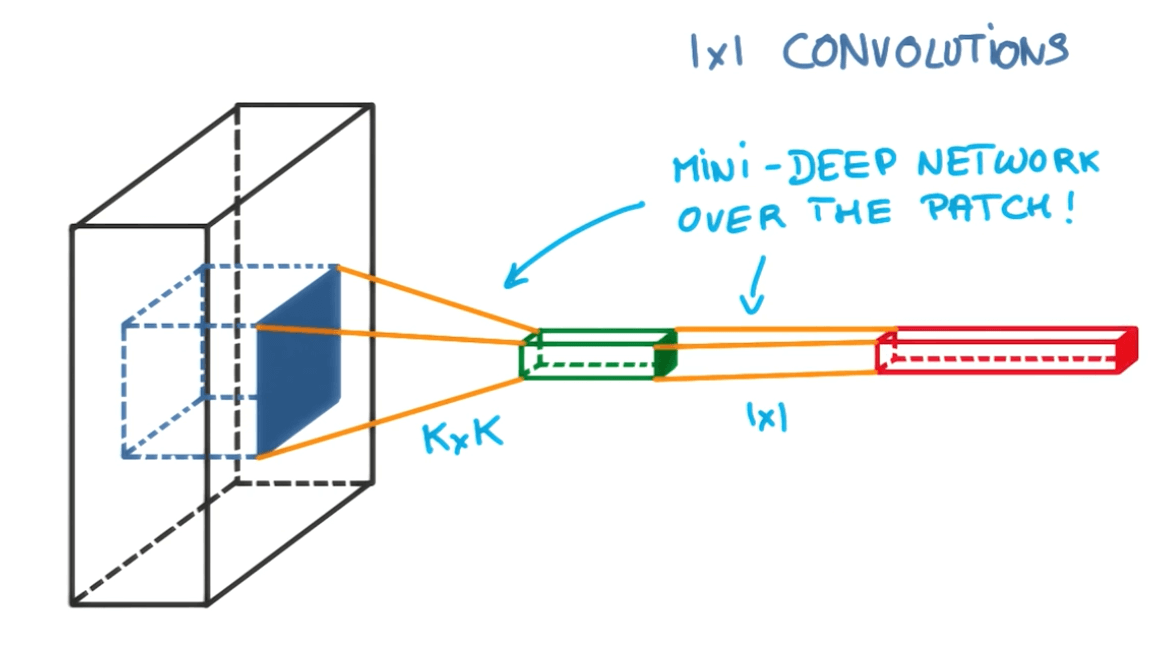Inception Module

• This is like an ensemble of methods.
•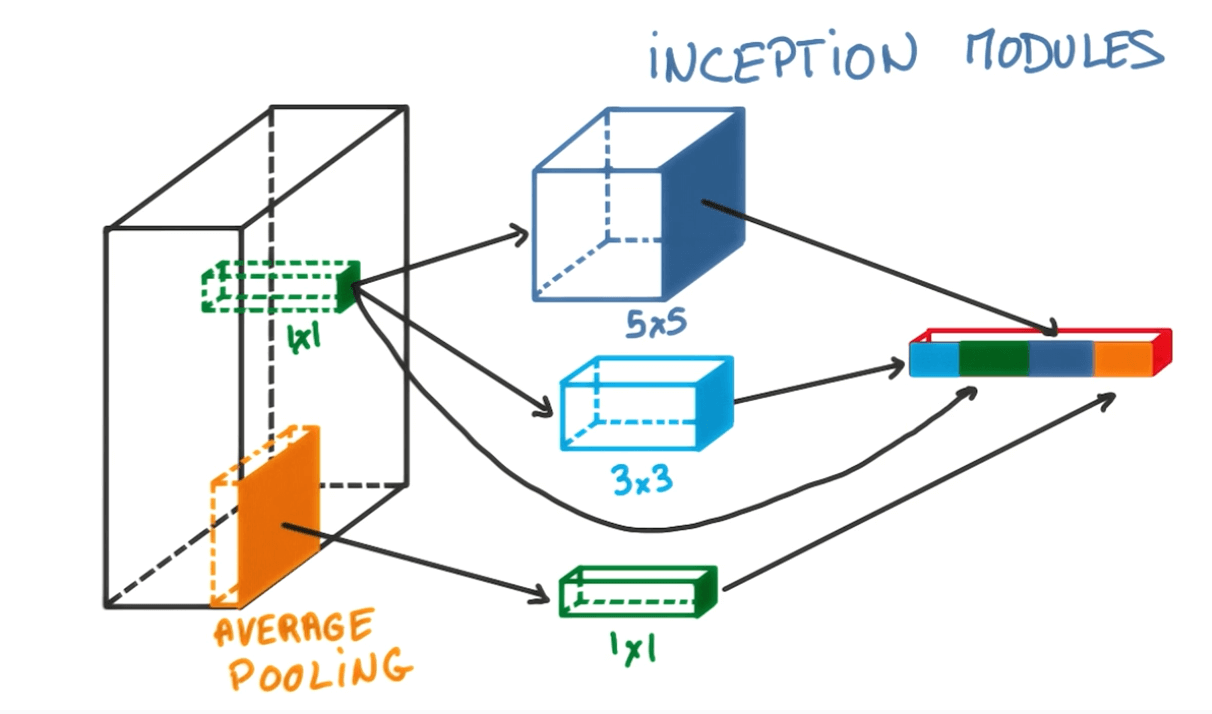Evaluation of results

• We can use accuracy to evaluate the predicted values and our labels.
• But a better method would be to use the top-1 and top-5 errors.

Progress in Convs (in order of lower top-1 and top-5 errors)

• LeNet-5
• AlexNet
• ZFNet
• VGGNet